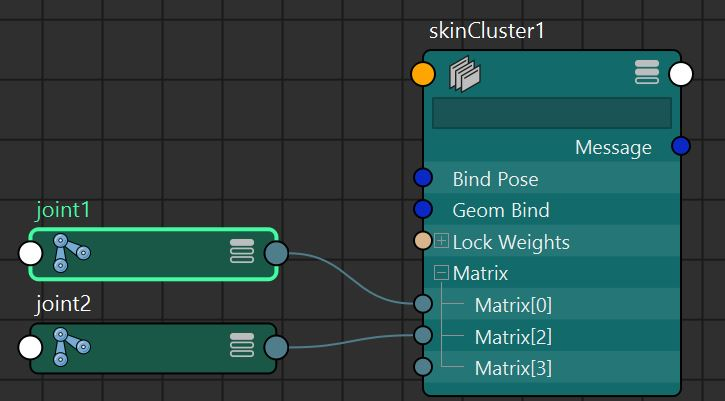# Maya: look up array attribute connections# MEL

Consider this node and connections to the array (multi) attribute `.matrix`:MEL command to list every DG nodes connected to a multi attribute (a.k.a array attribute):

```string \$joint_names[] = `listConnections (\$skincluster+".matrix")`;
// example output: {'joint1', 'joint2'}
```

Now here is how to list the "logical" indices of an array attribute that are connected to a node:

```int \$joint_indices[] = `getAttr -multiIndices (\$skincluster+".matrix")`;
// example output: {0, 2}
```

Note: you may recall that array attributes can be sparse, for instance:

```    array_attribute : [ joint1,  joint2]
Logical indices    : {      0,       2}
Physical indices   : {      0,       1}
```

## Summary

This is how to transfer the connections of a multi (i.e. array) attribute from one Maya node to another:
```string \$joint_names[] = `listConnections (\$source_skincluster+".matrix")`;
int \$joint_indices[] = `getAttr -multiIndices (\$source_skincluster+".matrix")`;

// Loop through the nodes connected to the attribute '\$source_skincluster+".matrix[]"'
for( \$i = 0; \$i < size(\$joint_indices); ++\$i) {
int \$idx = \$joint_indices[\$i];
string \$name = \$joint_names[\$i];
// Reconnect joints to another skin cluster while preserving the original indices of the joints:
connectAttr (\$name+".worldMatrix") (\$destination_skincluster+".matrix["+\$idx+"]")
}
```

# Python 2.0 API

The above MEL code translates as follows:

```from maya.api.OpenMaya import *

# get_MObject() and get_MPlug() are utilities I define below
obj = get_MObject("skinCluster1")
plug = get_MPlug(obj, "matrix")
for i in range(plug.numElements()):
plug_element = plug.elementByPhysicalIndex(i)
print( "name: " + plug_element.name() )
print( "logical index: " + str(plug_element.logicalIndex()) )
print( "physical index: " + str(i) )
print( "" )
```

Output:

```# name: skinCluster1.matrix
# logical index: 0
# physical index: 0
#
# name: skinCluster1.matrix
# logical index: 2
# physical index: 1
```

```from maya.api.OpenMaya import *

def get_MObject(node_name):
if isinstance(node_name, MObject):
return node_name

if isinstance(node_name, MDagPath):
return node_name.node()

slist = MSelectionList()
try:
except RuntimeError:
assert False, 'The node: "'+node_name+'" could not be found.'

matches = slist.length()
assert (matches == 1), 'Multiple nodes found for the same name: '+node_name

obj = slist.getDependNode(0)
return obj
# -----------------

def get_MPlug(obj, attribute):
if not isinstance(obj, MObject):
assert False, "expected MObject"
dep = MFnDependencyNode(obj)
plug = MPlug()
try:
plug = dep.findPlug( attribute, True)
except RuntimeError:
assert False, 'The attribute: "'+attribute+'" could not be found.'

assert (not plug.isNull), 'The attribute: "'+attribute+'" could not be found.'
return plug
# -----------------
```